# How to Create User Defined Function in Microsoft Excel

In this article, we are going to learn how to create user defined functions in Microsoft Excel by using the VBA.

User Defined Function:- Microsoft Excel is already having lot of functions, but still everybody has different requirements, situation, we can create our own function as per the requirement that is called User Defined Function. We can use User Defined function like other functions  in Excel.

Below are the topics for which we will create the user defined function:

1). How to count the number of words in Cell or range?

2). How to extract a word from a Sentence or Cell in Excel?

3). How to create the formula for ISO?

4). How to Know the Worksheet and Workbook name using the VBA?

5). How to extract the first and last word from a cell in Excel?

How to create the user defined function to count the number of words in Cell or Range?

We have data in sheet 1 in which we have some addresses so we want to count the words in a cell or a range by creating the user defined function through VBA in Excel.

• Open VBA Page and press the key Alt+F11.
• Insert a module.

Write the below mentioned code:

```Function WORDSCOUNT(rRange As Range) As Long
Dim rCell As Range
Dim Count As Long
For Each rCellInrRange
lCount = lCount + Len(Trim(rCell)) - Len(Replace(Trim(rCell), " ", "")) + 1
Next rCell
WORDSCOUNT = lCount
End Function
```

Code Explanations: - To make the user defined function we start the code to function name and define the variables. We have used “For Each loops” in the code to count the words in the range.

How to use this function in Excel?

To use this function, follow below given steps:-

• Go to Excel Sheet.
• To count the words for a cell, enter the formula in cell D7.
• =WORDSCOUNT(C7), cell C7 is the cell in which we want to calculate the words.
• The function will return 6, which means cell C7 contains 6 words.
• To do the same calculation for the rest of the cells, copy the same formula and paste in the range.
• To count the words in the range, use the formula as =WORDSCOUNT(C7:C16), and press Enter.
• The function will return the count of the words.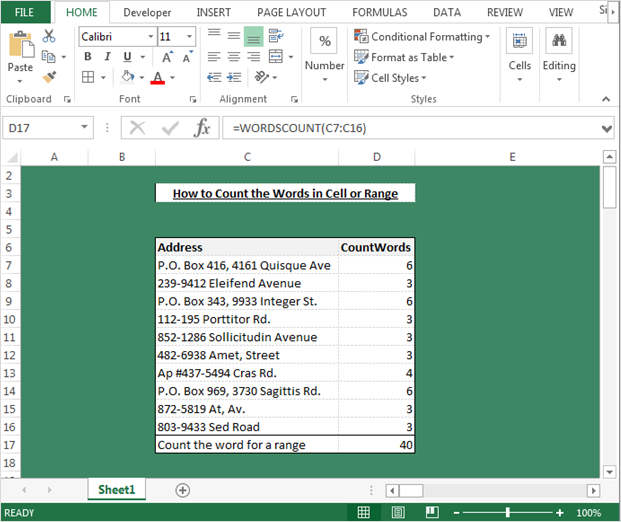Note:- This UDF will be helpful to count the words in a range or in a single cell.

Now we will write the code to count the word by using the specified delimiter (,). Follow below given steps:-

```Function SEPARATECOUNTWORDS(rRange As Range, Optional separator As Variant) As Long
Dim rCell As Range
Dim Count As Long
If IsMissing(separator) Then
separator = ","
End If
For Each rCellInrRange
lCount = lCount + Len(Trim(rCell)) - Len(Replace(Trim(rCell), separator, ""))
Next rCell
SEPARATECOUNTWORDS = lCount
End Function
```

To use this function, follow below given steps:-

• Go to Excel Sheet.
• To count the specific delimiters in the word, we will user this defined function.
• =SEPARATECOUNTWORDS(C7) and  press Enter.
• The function will return the count of the specific delimiters.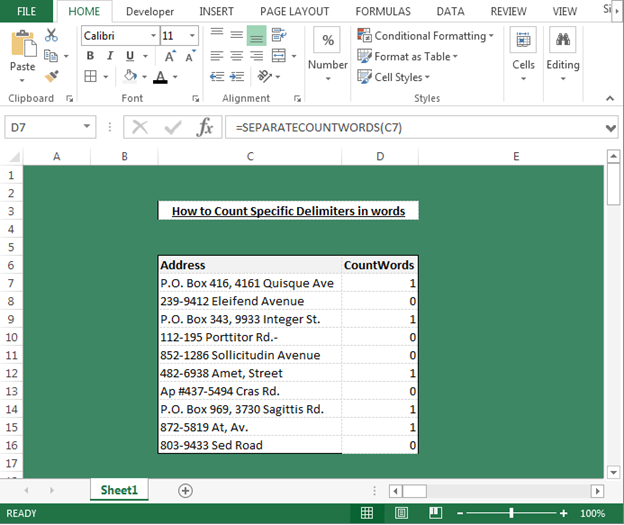How to extract a word from a sentence or cell in Microsoft Excel using the VBA?

We have data in sheet1. In which we have some addresses so we want to extract the words from a sentence or cell or a range by creating the user defined function through VBA in Excel.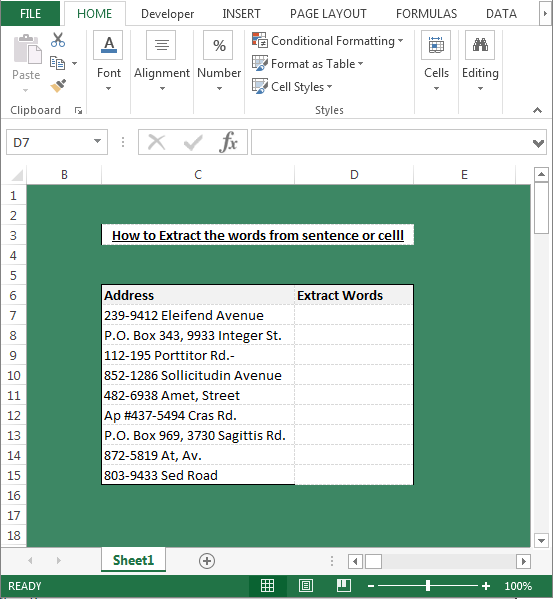• Open VBA Page and press the key Alt+F11.
• Insert a module.
• Write the below mentioned code:-
```Function GETWORD(Text As Variant, N As Integer, Optional Delimiter As Variant) As String
If IsMissing(Delimiter) Then
Delimiter = " "
End If
GETWORD = Split(Text, Delimiter)(N - 1)
End Function
```

Code Explanation:- In the above mentioned code, we have mentioned function name with the variables. And then we had defined the criteria to extract the word from sentence or cell.

Now we will learn how to use this formula. Follow below given steps:-

• Go to Excel Sheet.
• Use this formula in cell D7.
• =GETWORD(C7,2) and press Enter.
• The function will return second word from the cell because in formula we had mentioned for 2nd number’s word. If you want to retrieve the word which is placed at 3rd position, you need to change the number from 2 to 3 in formula.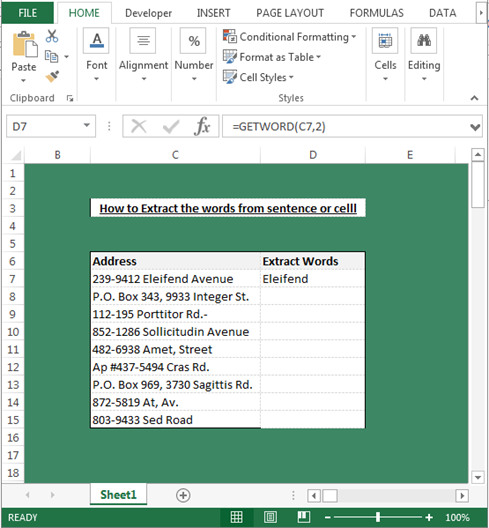How to create ISO Week number formula in Microsoft Excel using the VBA?

We will learn how we can create ISO week number formula in Excel with this UDF. This function we will use to identify that the mentioned date belongs to which week number of the year.

We have a list of date in the sheet, and in the second column, we want to retrieve the week numbers.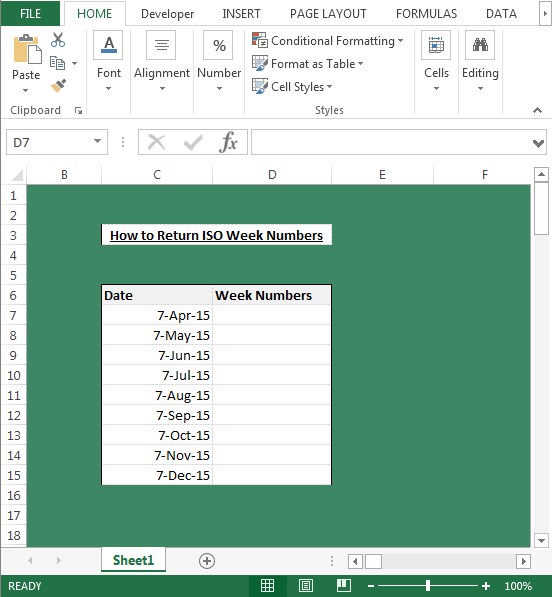To create the UDF for this requirement, follow below given steps:-

• Open VBA Page and press the key Alt+F11.
• Insert a module.
• Write the below mentioned code:-
```Function ISOWEEKNUMBER(Indate As Date) As Long
Dim Dt As Date
Dt = DateSerial(Year(Indate - Weekday(Indate - 1) + 4), 1, 3)
ISOWEEKNUMBER = Int((Indate - Dt + Weekday(Dt) + 5) / 7)
End Function
```

Code Explanation:- :- In the above code, we have mentioned function name with the variables. And then we had set the date value and then we had defined the “ISOWEENUMBER” function’s criteria.

How we can use this function in our Excel file?

• Go to Excel Sheet.
• Enter the formula in cell D7.
• =ISOWEEKNUMBER(C7), and press Enter.
• The function will return the week for the entered date in the cell. Now to retrieve the week number for the each date, copy the same formula in range.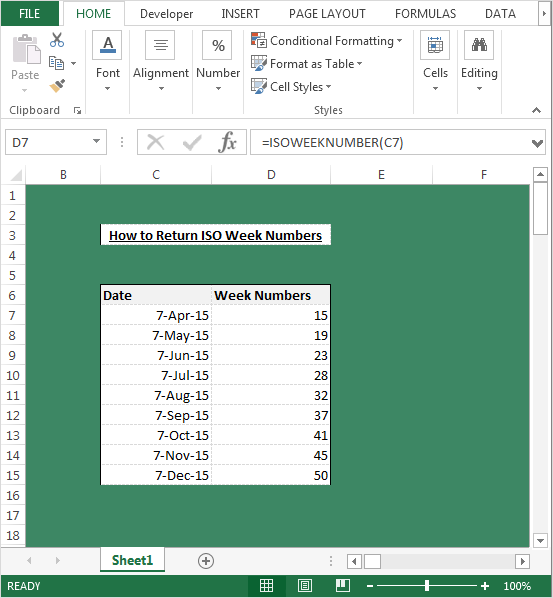Now we will learn how to return the ISO standards start of the year in Excel- First Monday of the Year.

This function will basically check that 1st Monday of the year is going to  fall on which date and then it will start to calculate the number of weeks from that date. Let’s see how we can create the UDF for this requirement.• Open VBA Page and press the key Alt+F11.
• Insert a module.
• Write the below mentioned code:-
```Function ISOSTYR(Year As Integer) As Date
Dim WD As Integer
Dim NY As Date
NY = DateSerial(Year, 1, 1)
WD = (NY - 2) Mod 7
If WD < 4 Then
ISOSTYR = NY - WD
Else
ISOSTYR = NY - WD + 7
End If
End Function
```

Code Explanation: - In the above code, we have mentioned function name with the variables. And, then we had set the criteria for the variables and then we had defined the formula input.

You just need to provide the year 2001 in this format and the formula will give you 1st Monday of the year.

Now we will learn how to use the UDF in Excel file. Follow below given steps:-

• Go to Excel Sheet.
• Enter the formula in cell D7.
• =ISOSTYR(C7) and press Enter.
• The function will return the date of the 1st Monday of the New Year’s first week.
• To return the date of the 1st Monday of the New Year’s first week, copy the same formula and paste in the range.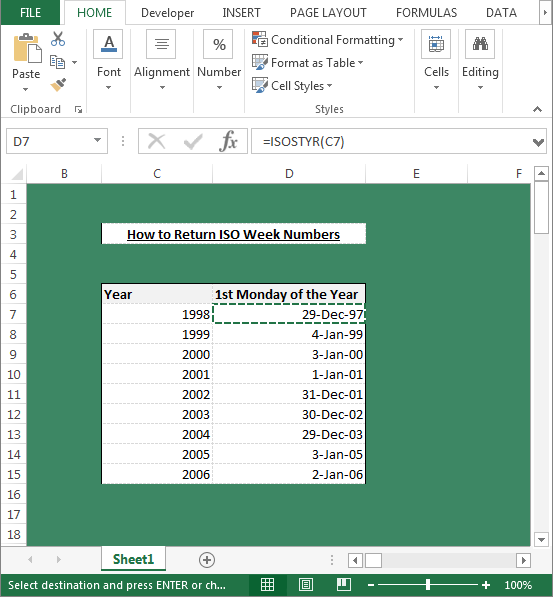How to know the Worksheet and Workbook name by using the VBA in Microsoft Excel?

Follow below given code and steps:-

• Open VBA Page and press the key Alt+F11.
• Insert a module.
• Write the below mentioned code:-
```Function Worksheetname()
Worksheetname = Range("A1").Parent.Name
End Function
```

Code Explanation:- In the above code, we have mentioned function name and then we had defined how to know the sheet name.

To use this formula, you just need to enter the formula in any cell in this way: -=Worksheetname(). The function will return the sheet name.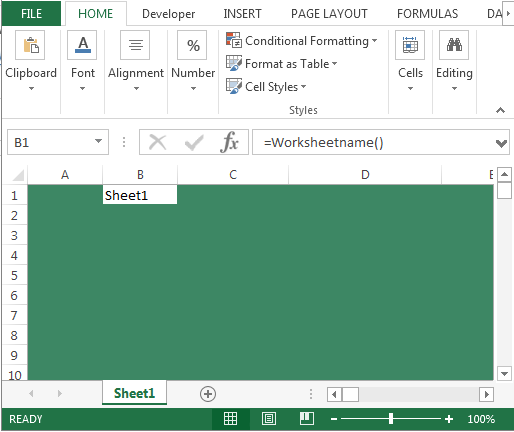To create the function for Workbook name, follow below given steps and code:-

• Open VBA Page press the key Alt+F11.
• Insert a module.
• Write the below mentioned code:-
```Function Workbookname()
Workbookname = ThisWorkbook.Name
End Function

```

Code Explanation:- :- In the above code, we have mentioned function name and then we had defined how to get to know the workbook name.

To use this formula, you just need to enter the formula in any cell in this way: - =Workbookname(). The function will return the sheet name.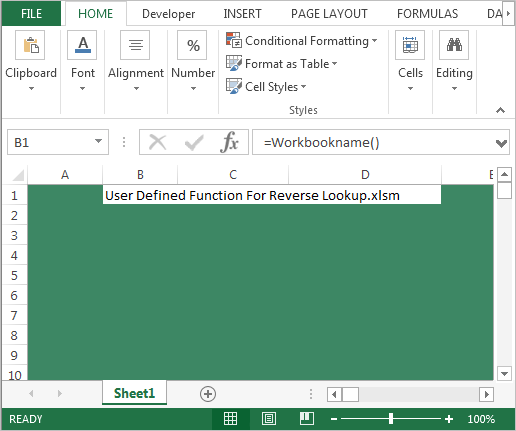How to extract the first and last word from a cell by using VBA in Microsoft Excel?

We have data in sheet1 in which we have some addresses so we want to extract the last and first word from a sentence or cell or a range by creating the user defined function through VBA in Excel.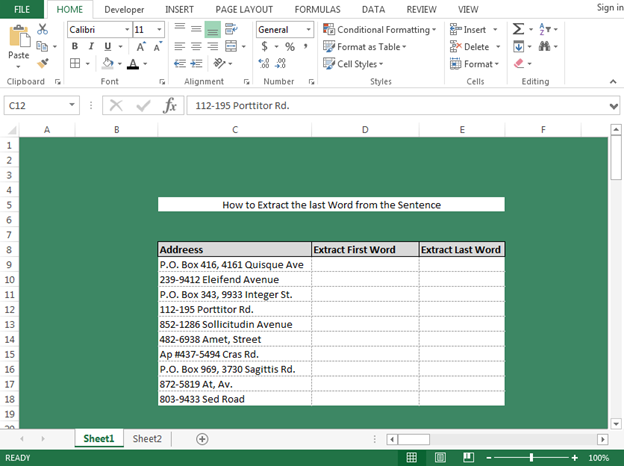First, we will write the function to extract the first word. Please follow below given steps:-

• Open VBA Page press the key Alt+F11.
• Insert a module

Write the below mentioned code:-

```Function GETFW(Text As String, Optional Separator As Variant)
Dim FW As String
If IsMissing(Separator) Then
Separator = " "
End If
FW = Left(Text, InStr(1, Text, Separator, vbTextCompare))
GETFW = Replace(FW, Separator, "")
End Function
```

Code Explanation: - In the above mentioned code, we have mentioned function name with the variables. And then we have defined the criteria to extract the word from sentence or cell.

Now we will learn how to use this formula. Follow below given steps:-

• Go to Excel Sheet.
• Use this formula in cell D9.
• =GETFW(C9) and press Enter.
• The function will return the first word from the data. Now, to retrieve the first word for all the cells, copy the same formula in the range.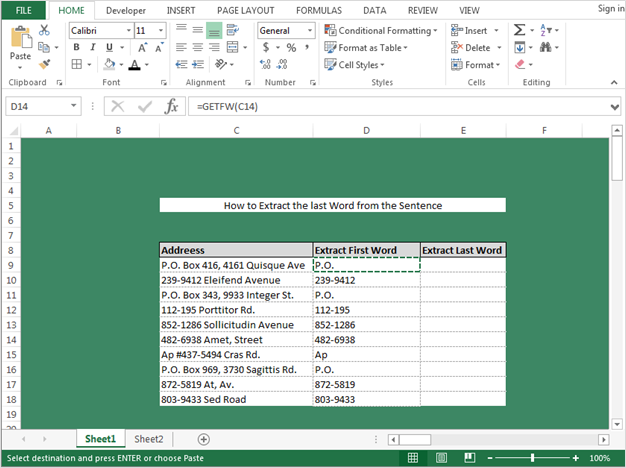Now we will write the code to extract the last word from the cell. Follow below mentioned code:-

• Open VBA Page press the key Alt+F11.
• Insert a module.
• Write the below mentioned code:-
```Function GETLW(Text As String, Optional Separator As Variant)
Dim LW As String
If IsMissing(Separator) Then
Separator = " "
End If
LW = StrReverse(Text)
LW = Left(lastword, InStr(1, LW, Separator, vbTextCompare))
GETLW = StrReverse(Replace(LW, Separator, ""))
End Function
```

Now we will learn how to use this formula. Follow below given steps:-

• Go to Excel Sheet.
• Use this formula in cell D9.
• =GETLW(C9) Press Enter.
• The function will return the last word from the data. Now, to retrieve the last word for all the cells, copy the same formula in the range.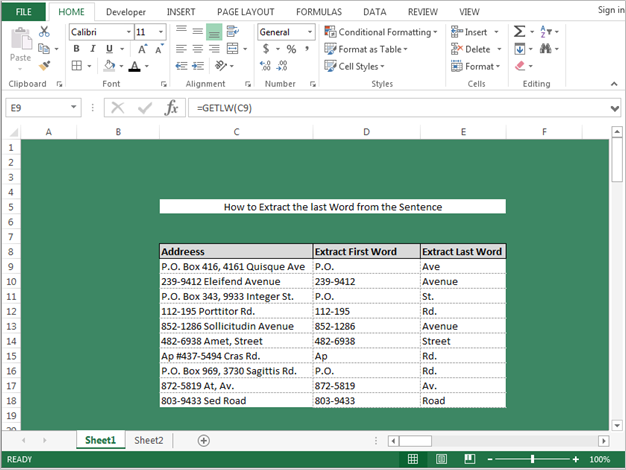These are the functions we can define through VBA and then can use it as formula of Excel. Also, we can create many more user defined function. Keep learning with us, we will come up with more tricky formulas.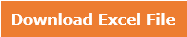Terms and Conditions of use

The applications/code on this site are distributed as is and without warranties or liability. In no event shall the owner of the copyrights, or the authors of the applications/code be liable for any loss of profit, any problems or any damage resulting from the use or evaluation of the applications/code.